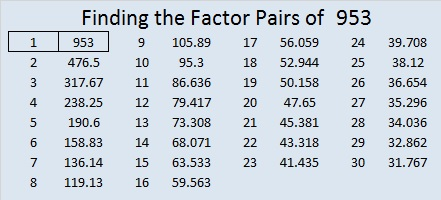# 953 and Level 2

This level 2 puzzle is only a tiny bit more difficult than a level 1 puzzle is. Start by finding the common factors of 4, 12, 40, and 28. The common factors are 1, 2, and 4, but 4 is the only one that works for the puzzle because we aren’t allowed to put factors like 14 or 28 in the top row. We are only allowed to write factors from 1 to 10 in the first column or the top row. Give this puzzle a try. I’m confident you can solve it!Print the puzzles or type the solution in this excel file: 10-factors-951-958

Prime number 953 is the sum of the 21 prime numbers from 7 to 89.

28² + 13² = 953, so 953 is the hypotenuse of a Pythagorean triple:
615-728-953 calculated from 28² – 13², 2(28)(13), 28² + 13²

953 is a palindrome in base 11 and base 28:
797 in BASE 11 because 7(11²) + 9(11¹) + 7(11⁰) = 953
161 in BASE 28 because 1(28²) + 6(28¹) + 1(28⁰) = 953

953 × 19 × 3 = 54321, making 953 its biggest prime factor. Thank you Stetson.edu for that fun fact.

• 952 is a prime number.
• Prime factorization: 952 is prime.
• The exponent of prime number 952 is 1. Adding 1 to that exponent we get (1 + 1) = 2. Therefore 952 has exactly 2 factors.
• Factors of 952: 1, 952
• Factor pairs: 952 = 1 × 952
• 952 has no square factors that allow its square root to be simplified. √952 ≈ 30.854497

How do we know that 952 is a prime number? If 952 were not a prime number, then it would be divisible by at least one prime number less than or equal to √952 ≈ 30.9. Since 952 cannot be divided evenly by 2, 3, 5, 7, 11, 13, 17, 19, 23, or 29, we know that 952 is a prime number.Here’s another way we know that 952 is a prime number: Since its last two digits divided by 4 leave a remainder of 1, and 28² + 13² = 952 with 28 and 13 having no common prime factors, 952 will be prime unless it is divisible by a prime number Pythagorean triple hypotenuse less than or equal to √952 ≈ 30.9. Since 952 is not divisible by 5, 13, 17, or 29, we know that 952 is a prime number.